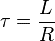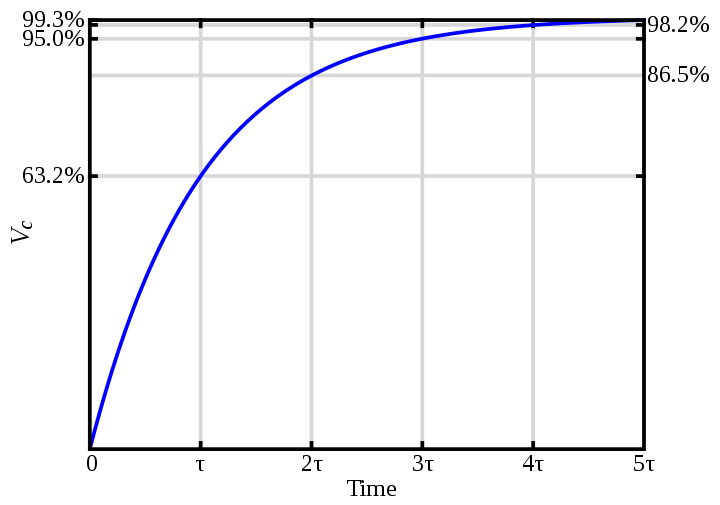The following is an explanation of commonly used terms in the motion control field.

Abbe Error - The angular error that occurs when the measuring point of interest is displaced from the actual measuring scale location.

Acceleration - Rate of change of velocity in g's(gravity constant) which is equal to 386 in/sec2 = 9.81 m/sec2.

Accuracy - is the difference in position between the actual position of the payload and the programmed position of the payload. It is measured in (inches or [microns]).

Air Gap - Distance required between the linear motor stationary and moving members.

Back EMF - Electro-Magnetic Force generated as the coil windings move through the magnetic field of the secondary. Back EMF constant is the ratio between mechanical motion components which may occur when standing or reversing direction.

Backlash - Deadband(lost motion) caused by clearances between mechanical motion components which may occur when starting or reversing direction.

Bandwidth - Frequency range in which a servo operates.

Closed Loop - Use of a feedback device to measure speed and or position.

Cogging - The tendency of some linear motors to move unplanned discrete distances. This effect is a result of varying magnetic forces along the length of the motor and platen.

Commutation - The sequence at which the controller switches the drive voltage/current among the various motor windings to ensure smooth linear motion of the motor. Commutation can be achieved through the use of brushes, hall effect sensors, or with a sinusoidal current.

Duty Cycle - Percentage of time during a specified time interval that power is applied to the motor. If time on is greater than 30 secs the duty cycle is 100%.

Efficiency - Ratio of output power to input power.

Electrical Time Constant - is the amount of time it takes the current in the motor to build up to 63.2% of its final current. In the linear motors and actuators, the electrical time constant is calculated by:, (L = inductance, R = resistance)Encoder - A position feedback device that outputs an analog or digital signal proportional to position.

Flatness - is the variation of the vertical position of the stage as it travels down its length. Better flatness can be achieved using the AB and XR stages. It is measured in (in/ft or [microns/m])

Forcer Linear stepper motor component that contains the coil assembly.

Force Ripple - The periodic force variation that occurs when the motor is in operation.

Full Step - Longest distance that the stepper motor moves during one pulse.

Half Effect Sensor(HES) - A semiconductor chip that senses magnetic flux changes when passing over magnets. Typically a Hall Effect Sensor is used for commutation in brushless motors.

Home Position - Unique position used as the initialization location from which all other porgrammable locations are referenced. Also known as the reference marker.

How Big Is Micron - Use the example and illustration bellow to better understand exactly how big a micron is. The scale of the diagram is 1000 to 1 ratio.

The thickness of a standard piece of paper measures 0.004 inch (0.1016 mm).

In comparison, a circle of 0.001 inch (0.0254 mm) diameter is shown. Note how much smaller it is than the piece of paper. 1 micron is 1/100 of the paper thickness.

A circle with a diameter of 1 micron is shown. A micron measures 0.000039 inch (0.001 mm) diameter is shown. Note the extremely small size of this circle compared to the piece of paper.Indexer - A programmable motion controller that sends step or step and direction signals to the linear stepper motor driver.

Laser Interferometer - Feedback device used to measure the distance between the incident and reflected light beam. Provides the highest degree of resolution and accuracy.

Linear Motor - type of motor that converts electric current into direct linear motion without ball screws, gears, or other mechanical transmission systems.

Mapping - Method used to calibrate or compensate for repeatable system errors.

Microstep - A finer unit of displacement that is achieved by proportioning the current in each phase of the linear stepper motor. It provides smoother motion and higher resolution.

Orthogonality - Measure of squareness(90 degree relationship) between motion axes. The value of error is typically expressed in arc-seconds.

Platen - The long stationary component of the linear stepper motor.

Primary - The linear motor component that contains the coil assembly.

Repeatability - The discrepancy between two successive approaches of a motor to a target position. Approaching the target position from the same direction twice and determining the discrepancy between them provides unidirectional repeatability. Approaching the target from two different directions and determining the discrepancy between them provides bidirectional repeatability.

Resolution - The smallest displacement that a motor is able to move or the smallest displacement an encoder is able to detect.

Rotary Motor - Type of motor that converts electric current into rotational motion.

Secondary - Linear motor component that contains the steel and permanent magnets or aluminium plates.

Settling Time - The time required for the system to settle within a finite error band of its final position after a commanded move.

Servo - An electric motor used in feedback control of the mechanical device for closed loop systems.

Stepper Motor - A motor that moves in predetermined steps. The stepper motor's position is determined by the number of step pulses that are supplied to the motor. Typically, this type of motor operates as an open loop system. Although a position verification sensor is available to provide closed loop operation.

Stiffness - Ability of an object to resist displacement. A measure of deflection for a positioning axis under load. Expressed in pound per inch.

Straightness - is the variation of the horizontal position of the stage as it travels down its length. Better flatness can be achieved using the AB and XR stages. It is measured in (in/ft or [microns/m])

Understanding Linear Encoder Resolution -

Typical Linear Encoder Resolutions (in order from lowest to highest):
• 5-micron encoder - A 5-micron encoder resolution means that over 1 meter of travel there are 200,000 different points that the encoder can read. Each point on the encoder scale/tape is exactly 5 micron (0.005 mm) away from the next.
• 1-micron encoder – A 1-micron encoder resolution means that over 1 meter of travel there are 1 million different points that the encoder can read. Each point on the encoder scale/tape is exactly 1 micron (0.001 mm) away from the next.
• 0.1-micron encoder - A 0.1-micron encoder resolution means that over 1 meter of travel there are 10 million different points that the encoder can read. Each point on the encoder scale/tape is exactly 0.1 micron (0.0001 mm) away from the next.

Note: The final positioning resolution of a properly tuned closed loop servo system with an encoder is typically ±2 encoder counts. So with a system that has a 1 micron resolution encoder on it, you can expect to achieve a final position that is within ±2 microns of the desired position.

Velocity Ripple - periodic velocity fluctuation that occurs when the motor is in operation.